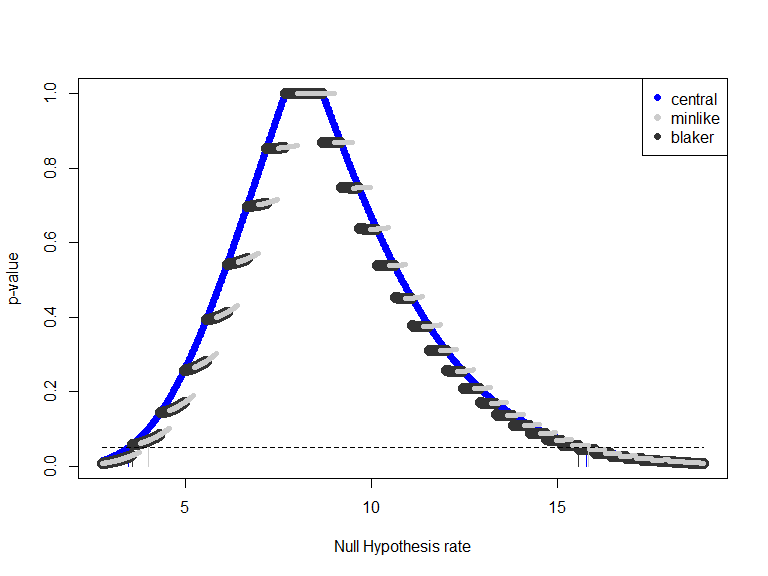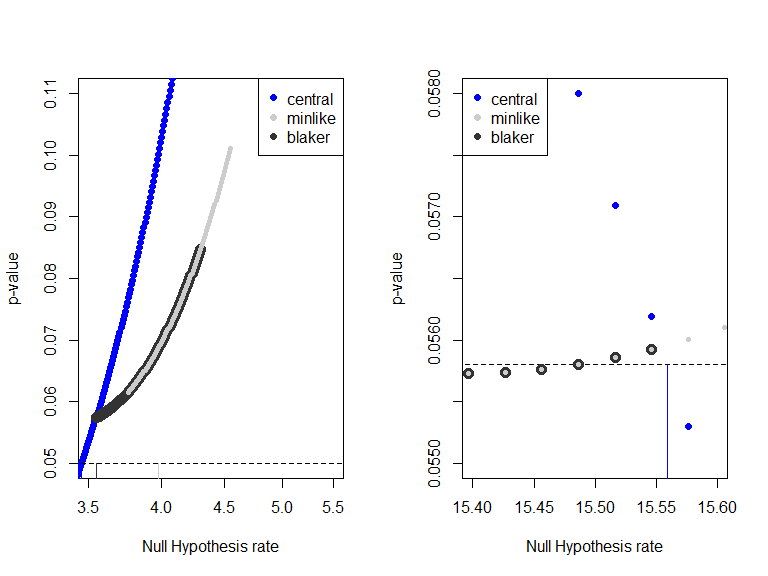1. Calculation of Two-sided Poisson Confidence Intervals

Klaschka and Reiczigel (2020) gave better algorithms for calculating the Blaker and minlike (i.e., Sterne) two-sided confidence intervals. They focus on the discontinuity points. Borrowing from those some of the main ideas in that paper, but using different notation, we create an algorithm to calculate the $$100(1-\alpha)$$% two-sided Poisson confidence intervals. Checks of this algorithm show that it gets nearly identical results as Klaschka and Reiczigel (2020) in all situations that were checked.

Let $$X \sim Poisson(\theta)$$. Let a general two-sided p-value for testing $$H_0: \theta=\theta_0$$ versus $$H_1: \theta \neq \theta_0$$ at $$X=x$$ be $$p_T(x,\theta_0)$$. Let the associated two-sided $$100(1-\alpha)$$% confidence interval be $\left( L_T(x, 1-\alpha), U_T(x, 1-\alpha) \right),$ where $L_T(x, 1-\alpha) = \sup \left\{ \theta: \mbox{ for all } \theta_0 \leq \theta \mbox{ then } p_T(x, \theta_0) \leq \alpha \right\}$ and $U_T(x, 1-\alpha) = \inf \left\{ \theta: \mbox{ for all } \theta_0 \geq \theta, \mbox{ then } p_T(x, \theta_0) \leq \alpha \right\}$.

2. Sterne (i.e., minlike) Method

2.1 Overview of Algorithm

Let $$f(i; \theta)=Pr[ X=i | \theta]$$ be the Poisson probability mass function at $$X=i$$. Then the ‘minlike’ p-value for testing $$H_0: \theta=\theta_0$$ is $p_m(x, \theta_0) = \sum_{i: f(i;\theta_0) \leq f(x;\theta_0)} f(i;\theta_0).$ We can write $$p_m$$ in a format that is conducive for calculating the confidence interval. First, define $\tilde{\theta}_{a,b}$ as the geometric mean of $$a+1, a+2,\ldots, b$$, where $$a<b$$ and both are non-negative integers. Then we can show that $$f(a; \tilde{\theta}_{a,b}) = f(b, \tilde{\theta}_{a,b})$$.

Writing out $$f(i;\tilde{\theta}_{a,b})$$ for $$i=a,b$$, and setting them equal gives,

$\frac{\tilde{\theta}_{a,b}^{a} e^{-\tilde{\theta}_{a,b}} }{a!} = \frac{\tilde{\theta}_{a,b}^{b} e^{-\tilde{\theta}_{a,b}} }{b!},$ and solving for $$\tilde{\theta}_{a,b}$$ gives the definition of that geometric mean, $\tilde{\theta}_{a,b} = \exp \left( \frac{1}{b-a} \sum_{i=a+1}^{a+(b-a)} \log(i) \right).$ Thus, for $$x \geq 2$$ and $$2 \leq j \leq x$$, we have that for $$\tilde{\theta}_{x-j,x} \leq \theta_0 < \tilde{\theta}_{x-j+1,x}$$ we have $f(x-j;\theta_0) \leq f(x; \theta_0) < f(x-j+1;\theta_0).$ So that for $$\tilde{\theta}_{x-j,x} \leq \theta_0 < \tilde{\theta}_{x-j+1,x}$$, we have $p_m(x;\theta_0) = F(x-j;\theta_0) + \bar{F}(x;\theta_0)= 1 - \sum_{i=x-j+1}^{x-1} f(i;\theta_0),$ where $$F(x;\theta) = \sum_{i=0}^{x} f(i;\theta)$$ and $$\bar{F}(x;\theta) = \sum_{i=x}^{\infty} f(i;\theta) = 1- \sum_{i=0}^{x-1} f(i;\theta)$$, with $$F(x;\theta)=0$$ when $$x<0$$.

Thus, $p_m(x;\theta_0) = \left\{ \begin{array}{ll} F(x-j;\theta_0) + \bar{F}(x;\theta_0) & \mbox{ if } \tilde{\theta}_{x-j,x} \leq \theta_0 < \tilde{\theta}_{x-j+1,x}, j =2,...,x+1 \\ 1 & \mbox{ if } \tilde{\theta}_{x-2,x} \leq \theta_0 \leq \tilde{\theta}_{x,x+1} \\ F(x;\theta_0) + \bar{F}(x+j+1;\theta_0) & \mbox{ if } \tilde{\theta}_{x,x+j} < \theta_0 \leq \tilde{\theta}_{x,x+j+1}, j \geq 1 \\ \end{array} \right.$ Consider the piecewise continuous part where $$\tilde{\theta}_{x-j,x} \leq \theta_0 < \tilde{x-j+1,x}$$. At $$\theta_0= \tilde{\theta}_{x-j,x}$$, then $$f(x-j;\theta_0)=f(x;\theta_0)$$, as $$\theta_0$$ increases then $$f(x-j;\theta_0)<f(x;\theta_0)$$ and no other value is added to the $$F(x-j;\theta_0)$$ part of the p-value until $$\theta_0=\tilde{x-j+1,x}$$.
The p-value is piecewise continuous part where $$\tilde{\theta}_{x,x+j} < \theta_0 \leq \tilde{x,x+j+1}$$. At $$\theta_0= \tilde{\theta}_{x,x+j+1}$$, then $$f(x;\theta_0)=f(x+j+1;\theta_0)$$, as $$\theta_0$$ decreases then $$f(x;\theta_0)>f(x+j+1;\theta_0)$$ and no other value is added to the $$\bar{F}(x+j;\theta_0)$$ part of the p-value until $$\theta_0=\tilde{x,x+j}$$.

Using derivatives, we can show that during each piecewise continuous part of the p-value when $$\theta_0>x$$, the p-value function is increasing in $$\theta_0$$.
Consider the piecewise continuous part with $$p_m= F(a;\theta_0) + \bar{F}(b;\theta_0)$$ with $$a<b$$. First, rewrite $$p_m$$ as $p_m = \sum_{i=0}^{a} f(i;\theta_0) + 1 - \sum_{j=0}^{b-1} f(j;\theta_0)$ which simplifies to $p_m = 1 - \sum_{j=a+1}^{b-1} f(j;\theta_0).$ Now we take the derivative with respect to $$\theta_0$$. Notice that $\frac{\partial f(j;\theta)}{\partial \theta} = \frac{1}{j!} \frac{\partial \theta^j e^{-\theta}}{\partial \theta} = \frac{1}{j!} \left( j \theta^{j-1} e^{-\theta} - \theta^j e^{-\theta} \right)= f(j-1;\theta) - f(j;\theta)$ So that $\frac{ \partial p_m}{\partial \theta_0} = \frac{ \partial \left( 1 - \sum_{j=a+1}^{b-1} f(j;\theta_0) \right) }{\partial \theta_0}= - \sum_{j=a+1}^{b-1} \frac{ \partial f(j;\theta_0)}{\partial \theta_0} = \sum_{j=a+1}^{b-1} \left\{ f(j;\theta_0) - f(j-1;\theta_0) \right\} = f(b-1;\theta_0) - f(a; \theta_0).$

When $$a=x$$ and $$b=x+j+1$$, then when $$\tilde{\theta}_{x,x+j} < \theta_0 \leq \tilde{\theta}_{x,x+j+1}$$ and the derivative is $$f(x+j;\theta_0) - f(x;\theta_0).$$ Just below the lower end of the interval, $$f(x+j;\theta_0) =f(x;\theta_0)$$, then as $$\theta_0$$ increases $$f(x+j;\theta_0) >f(x;\theta_0)$$ so that the derivative is always positive, and the p-value is increasing.

So the upper confidence limit of the $$100(1-\alpha)$$% minlike CI is the largest $$j$$ such that $$p_m(x,\tilde{\theta}_{x,x+j+1})> \alpha$$.

For the lower confidence limit we conjecture that the piecewise continuous p-value function is monotonic within each continuous piece.

2.2 Compare Output to Function of Reiczigel

We compare our results to those of from the function of Reiczigel as referenced in Kaschka and Reiczigel (2020). The function was accessed in May 2021 at the URL referenced in Kaschka and Reiczigel (2020); however, the website was under re-construction in June 2021. I suggest going to https://www2.univet.hu/ and following links to J. Reiczigel’s homepage to find the function (or look at the Rmd file that created this vignette).

Compare Results
x conf level lower CL upper CL
Reiczigel 1 0.70 0.3566749 3.309751
Fay 1 0.70 0.3566749 3.309751
Reiczigel 0 0.95 0.0000000 3.764351
Fay 0 0.95 0.0000000 3.764351
Reiczigel 8 0.99 2.9061062 18.807287
Fay 8 0.99 2.9061062 18.807287
Reiczigel 8 0.90 4.5319366 14.512948
Fay 8 0.90 4.5319366 14.512948
Reiczigel 8 0.01 8.0000000 9.000000
Fay 8 0.01 8.0000000 9.000000

3. Blaker’s Method

3.1 Motivation for Blaker’s Method

Blaker’s p-values are (see e.g., Klaschka and Reiczigel, 2020, Section 3.1) $p_B(x;\theta_0) = \left\{ \begin{array}{ll} F(x; \theta_0) + \bar{F}(x_R; \theta_0) & \mbox{ if F(x;\theta_0) < \bar{F}(x;\theta_0)} \\ 1 & \mbox{ if F(x;\theta_0) = \bar{F}(x;\theta_0)} \\ F(x_L; \theta_0) + \bar{F}(x; \theta_0) & \mbox{ if F(x;\theta_0) > \bar{F}(x;\theta_0)} \\ \end{array} \right. ,$ where $$x_R = min \left\{ i: \bar{F}(i;\theta_0) \leq F(x; \theta_0) \right\}$$ and $$x_L = max \left\{ i: {F}(x;\theta_0) \leq \bar{F}(i; \theta_0) \right\}.$$

This p-values are also piecewise continuous, but the jumps are at different places.

Here is a comparison of the three ways of calculating the 95% two-sided p-values for $$x=8$$: the central method, the minlike method, and Blaker’s method.An important computational point is that Blaker’s p-values are not monotonic within each piecewise constant interval. To see this, we focus in on a two pieces of the previous graph.We conjecture that on the lower side (p-values with $$\theta_0< x$$) the piecewise continuous function is monotonicly increasing within each interval, while on the upper side (p-values with $$\theta_0>x$$) the piecewise continuous function decreases to a inflection point, then increases (see the graph). We can solve for the inflection point by taking the derivative of the p-value function.

For $$a<b$$, let $$\hat{\theta}_{a,b}$$ be the value $$\theta_0$$ such that $F(a;\theta_0) = \bar{F}(b;\theta_0).$ As with the ‘minlike’ method, for calculating the confidence intervals
it is useful to rewrite the p-value function in terms of the piecewise continuous
functions with jumps at $$\hat{\theta}_{a,b}$$ values.

$p_B(x;\theta_0) = \left\{ \begin{array}{ll} F(x-j; \theta_0) + \bar{F}(x; \theta_0) & \mbox{ if \hat{\theta}_{x-j,x} \leq \theta_0 < \hat{\theta}_{x-j+1,x}} \\ 1 & \mbox{ if \hat{\theta}_{x, x+1} \leq \theta_0 \leq \hat{\theta}_{x-1, x}} \\ F(x; \theta_0) + \bar{F}(x+j; \theta_0) & \mbox{ if \hat{\theta}_{x,x+j} < \theta_0 \leq \hat{\theta}_{x,x+j+1}} \\ \end{array} \right. .$

Using the results of the previous section, we see that the inflection point on the interval betweeen $$\hat{\theta}_{x,x+j}$$ and $$\hat{\theta}_{x,x+j+1}$$ is at $$\tilde{\theta}_{x,x+j}$$. Using these assumptions, we can solve for the confidence intervals at either the jump points or using uniroot function when necessary.

Checking the Function

Klaschka developed the BlakerCI R package, so we can check our results against that.

Compare Results
x conf level lower CL upper CL
Klaschka 0 0.7000 0.0000000 1.568120
Fay 0 0.7000 0.0000000 1.568120
Klaschka 1 0.9500 0.0512933 5.525705
Fay 1 0.9500 0.0512933 5.525705
Klaschka 8 0.9442 3.5501406 15.553791
Fay 8 0.9442 3.5501406 15.553791
Klaschka 8 0.9440 3.5501406 15.199944
Fay 8 0.9440 3.5501406 15.199944
Klaschka 8 0.9430 3.5501406 15.118012
Fay 8 0.9430 3.5501406 15.118014